# 故障树分析（FTA）### 建树符号

#### 事件符号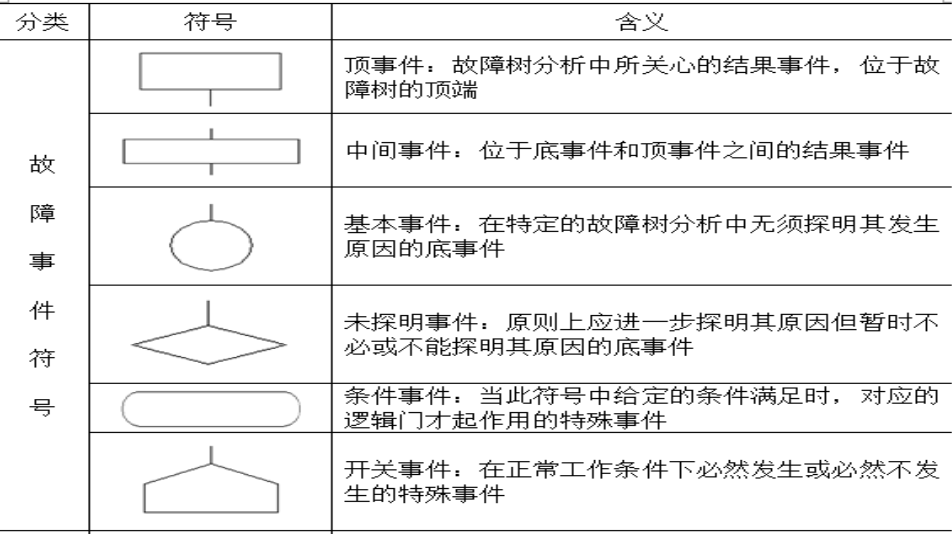#### 逻辑符号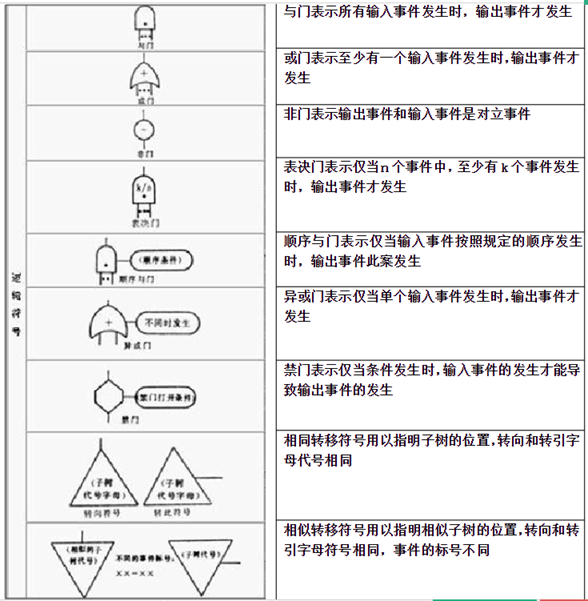#### 结构函数

• 底事件状态• 顶事件状态

可用与底事件有关的函数表示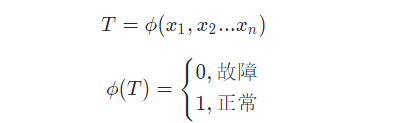• 计算

• 或门

\phi( T ) = \sum _ { i = 1 } ^ { n } { x _ i }

• 与门

\phi( T ) = \prod_{i = 1 }^{ n }{ x_i }

• 化简

T = x_1 ( x_1 + x_4 ) x_3 = x_1 x_3 + x_1 x_4 x_3 = x_1 x_3

• 最小割集

• 最小割集是指，是指让顶层事件发生的最少数量的底层事件集合
• 例：
• 顶层事件 T 可以表示为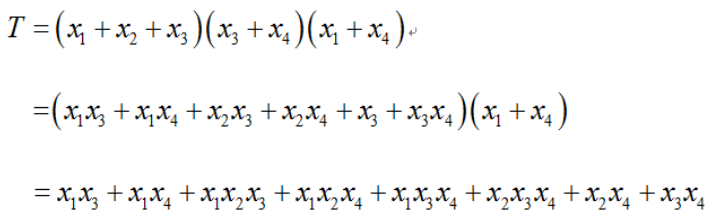• 化简为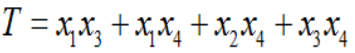• 根据表达式为或运算，则可知有 4 个最小割集，为：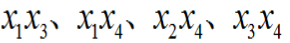• 路集：是割集的对偶集合，也即不触发发生顶层事件的底层事件集合

#### 顶层事件概率的计算

1. 将顶层事件表示为，最小割集的形式

T = \phi ( x_1 , x_2 ... x_n )

2. P 为计算事件的概率，则

P ( T ) = \phi ( P ( x_1 ) ,P ( x_2 )... P ( x_n ) )

#### 结构重要度计算

1. 设顶层事件 T，底层事件共 n 个

2. 计算底层事件 x 发生的情况下，顶层事件 T 发生的情况共 p 个

3. 计算底层事件 x 不发生的情况下，顶层事件 T 发生的情况共 q 个

4. 则事件 x 的结构重要度为

I(x) = \frac { 1 } { 2 ^ { n - 1 } } [ p - q ]

#### 概率重要度计算

1. 列出所有底事件状态组合与对应的顶事件状态
2. 找到其中其他条件相同，底事件 x 取 1 时顶事件 T 为 1，底事件 x 取 0 顶事件 T 为 0 的情况。
3. 计算概率
4. 例：
•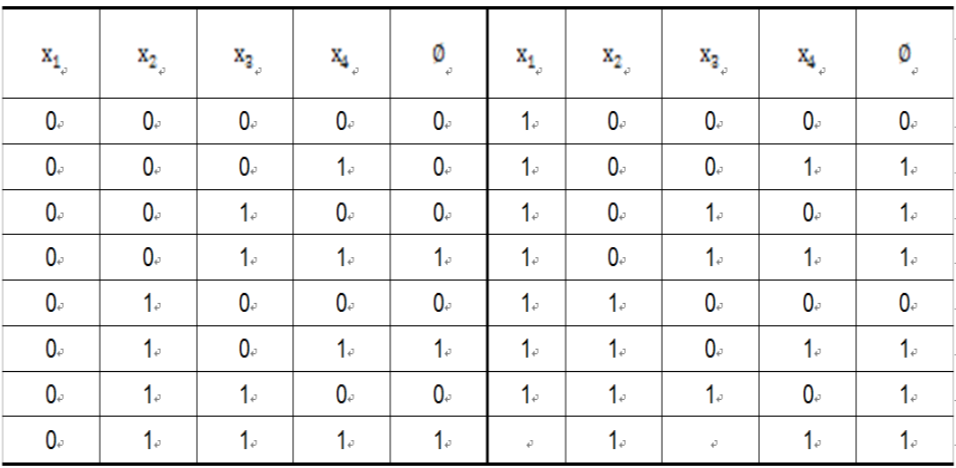• 组合 100110101110 满足条件
•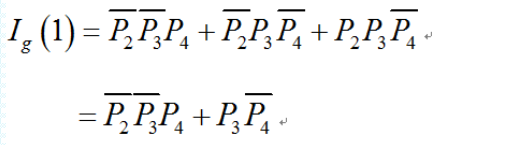#### 关键重要度计算

I_c(i) = \frac{P_i}{g(P)} I_g(i)

P_i为底事件i发生的概率

g(P)为顶事件发生的概率

I_g(i)为事件i的概率重要度